## Tuesday, September 20, 2005

### Pascal's Triangle and Pascal's Rule

Before we can proceed to the Binomial Theorem, we need to review a lemma that was introduced in the west by Blaise Pascal and which is today known as Pascal's Rule. The rule itself stems from an analysis of Pascal's Triangle.

Pascal's rule requires a basic understanding of the mathematics of permutations which concerns the problem of counting the number of different selections that can be made from a given set. For those who would like a review of the basic ideas of permutations and factorials, please start here. Some examples are below.

If we have only 1 element, then there is only 1 way of selecting 1 element. But if we have 2 elements, we have 2 different ways to select 1 element. If we have 15 elements, then there are 15 different ways to select 1 element and, it turns out, (15*14)/2 ways to select 2 elements. In other words, if we have n total elements and we want to select m of them, there are exactly n!/[m!(n-m)!] ways to select them where order doesn't matter. (See here for further explanation)

The formula for counting the number of ways to select m elements from a set of n total elements can be characterized by this shorthand notation: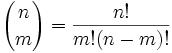In the case of selecting 2 items out of 15, we get the following: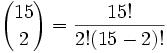Which gives us: 15!/(2!)(13)! = (15*14)/2.

With these ideas in mind, we can proceed to look at Pascal's Triangle. Pascal's Triangle is a way to characterize exponents such as (a + b)n

For example, the Triangle starts out as follows:

(n=0) 1
(n=1) 1 1
(n=2) 1 2 1
(n=3) 1 3 3 1
(n=4) 1 4 6 4 1
...

In each case, the number in the next row is equal to the sum of the two numbers above it (and if only one number is above it, then it is equal to this one number). We can keep on going as long as we like.

Let's see how this maps into the equation (a+b)n

(a + b)0 = (1)1
(a + b)1 = (1)a +(1) b
(a + b)2 = (1)a2 + (2)ab + (1)b2
(a + b)3 = (1)a3 + (3)a2b + (3)ab2 + (1)b3
(a + b)4 = (1)a4 + (4)a3b + (6)a2b2 + (4)ab3 + (1)b4

It turns out that each element of Pascal's Triangle can be built from an equation using the above equation. If we remember that 0! = 1, then we get the following

For n = 0, the single element is equal to: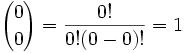For n = 1, each element can be described by the notation below: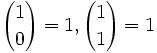For n = 2, each element can be described by: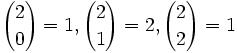For n = 3, each element can be described by: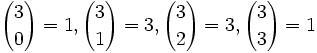And so on.

This is useful because it allows to pick any exponent n and generate the list of coefficients that make up its values.

For example, we know that the coefficients for n=7 will be: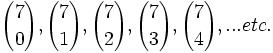So far so good. But wait a minute. When we were creating the triangle from step 1, we were adding each coefficient from the previous values. Is there a relationship here that can be captured in terms of the equation?

This is Pascal's Rule which is presented below.

Lemma: Pascal's Rule:(1)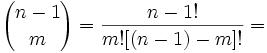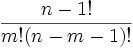(2)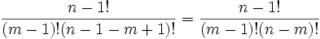(3) Now, the trick is to be able to add both of these values as fractions. This can be done based on the following equalities:

m! = (m)(m-1)!
(n-m)! = (n-m)(n-m-1)!

(4) Applying (3) to (1) and (2) gives us: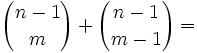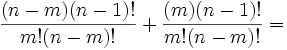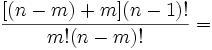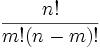QED

Scouse Rob said...

I'm a bit confused as to what would happen when n=m in the Pascal's Rule proof.

There is an (n-1-m)! in the denominator in step (1).

When n=m this will be (-1)!.

What is this defined as?

If it is 0 then there is a divide by zero in step 1.

Does there need to be a special case for:

(n)Choose(m), when m=n?

What is the definition of:

(A)Choose(B) when A<B? 0?

Sorry for the naive questions and poor notations.

Love the blog.

Rob

John said...

Pascal rule is defined for m,n both natural numbers, m is greater then or equal to n and m is greater then or equal to 1

in the case where n=m, the (n-1,m) binomial coefficient is not defined.